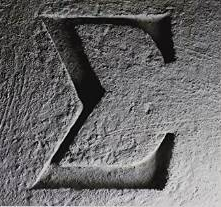# Verified Algorithm Analysis

## OverviewProof assistants, aka interactive theorem provers, are software systems for editing and mechanically checking mathematical definitions and proofs. Such mechanically checked texts (and the process of producing them) are called formalizations or verification. The last decade has produced a number of landmark formalizations in both mathematics (Gonthier’s formalization of the Feit-Thompson theorem using the Coq proof assistant and Hales’ formalization of his proof of the Kepler conjecture using the HOL and Isabelle/HOL proof assistants) and computer science (Leroy’s verification of a C compiler and Klein’s verification of an operating system kernel).

This research project uses the proof assistant Isabelle/HOL to support the formal analysis of the complexity of algorithms in addition to their functional correctness proofs. The two main aims are:

• Formalize the mathematical foundations required for quantitative program analysis.

• Create a library of formalized algorithms with verified proofs of functional correctness and complexity.The algorithms may be imperative, functional, deterministic or randomized.

An important subproject is the formalization of Tucker’s proof of Smale’s 14th problem that the Lorenz attractor is chaotic. This requires a formalization of ODEs and verified algorithms for their numeric analysis.

Funding: DFG Koselleck Project, 11/2015–11/2020

## Summary of Results

### Algorithms

We have formalized a framework for amortized analysis of functional data structures and have applied it to many standard examples as well as to the not so widely know general balanced trees by Andersson which we have named root-balanced.

We have verified the analysis of a number of classical algorithms for the list update problem, including the best known randomized one.

We have verified the correctness and complexity of the Edmonds-Karp algorithm for computing the maximum flow in a network.

We formalized the Akra-Bazzi method, a generalisation of the well-known Master Theorem, for analysing the complexity of Divide and Conquer algorithms.

We formally analyzed sorting algorithms: the number of comparisons in QuickSort, the lower bound on comparison-based sorting algorithms and the expected shape of random binary search trees.

We formalized Gröbner bases with the essentials of the theory (polynomial reduction, S-polynomials, Buchberger’s algorithm, Buchberger’s criteria for avoiding useless pairs) as well as more advanced features like reduced Gröbner bases. Particular highlights are the first-time formalization of Faugère’s matrix-based F4 algorithm and the fact that the entire theory is formulated for modules and submodules rather than rings and ideals.

### Smale’s 14th Problem

We have formalized foundations for reasoning about dynamical systems (such as the Lorenz attractor), in particular the notions of flow and Poincaré map.

We have verified rigorous numerical algorithms for solving ODEs and applied them to compute an enclosure the Lorenz attractor and formally verify the numerical part of Tucker’s proof of Smale’s 14th problem.

## Bibliography

1. Peter Lammich and S. Reza Sefidgar. Formalizing the Edmonds-Karp Algorithm. In ITP 2016, LNCS, 2016.
2. Tobias Nipkow. Amortized Complexity Verified. In ITP 2015, LNCS, 2015.
3. Maximilian P.L. Haslbeck, Tobias Nipkow. Verified Analysis of List Update Algorithms. In FSTTCS 2016.
4. Tobias Nipkow. Verified Root-Balanced Trees. In Asian Symposium on Programming Languages and Systems, APLAS 2017, LNCS, 2017.
5. Manuel Eberl. Proving Divide and Conquer Complexities in Isabelle/HOL. In Journal of Automated Reasoning 58(4), 2017.
6. Manuel Eberl. The number of comparisons in QuickSort. In Archive of Formal Proofs, 2017.
7. Manuel Eberl. Lower bound on comparison-based sorting algorithms. In Archive of Formal Proofs, 2017.
8. Manuel Eberl. Expected Shape of Random Binary Search Trees. In Archive of Formal Proofs, 2017.
9. Fabian Immler and Christoph Traut. The Flow of ODEs - Formalization of Variational Equation and Poincaré Map. In Journal of Automated Reasoning (2018).
10. Fabian Immler. Verified Reachability Analysis of Continuous Systems. In Tools and Algorithms for the Construction and Analysis of Systems, TACAS 2015.
11. Fabian Immler. A Verified Enclosure for the Lorenz Attractor (Rough Diamond). In Interactive Theorem Proving, ITP 2015.
12. Fabian Immler. A Verified ODE Solver and the Lorenz Attractor. In Journal of Automated Reasoning (2018) 61.
13. Alexander Maletzky and Fabian Immler. Gröbner Bases of Modules and Faugère’s F4 Algorithm in Isabelle/HOL. Extended version of paper accepted at CICM 2018.